Next: 4-Counter Geometry and the Complex Polarization Up: Measuring the penetration depth with TF-SR Previous: The Relaxation Function

# Modelling The Asymmetry Spectrum of the Vortex State

In terms of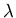, the experimental raw asymmetry for TF-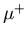SR analysis of the vortex state in an isotropic type-II superconductor can be modelled assuming that the contribution to A(t) from a particular point in the flux lattice is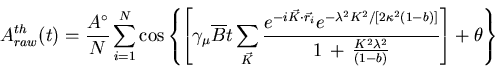(35)

where I have combined Eqs. (3.9), (3.27) and (3.31). Eq. (3.35) applies to an ideal flux lattice. To account for smearing of the field distribution due to flux-lattice disorder and nuclear dipolar fields, Eq. (3.35) must be convoluted with a gaussian distribution in the form of Eq. (3.34):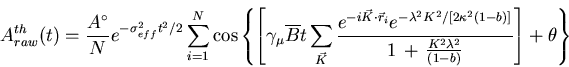(36)

Assuming that the flux-line lattice is composed of equilateral triangles as depicted in Fig. 3.1(a), an arbitrary reciprocal-lattice vector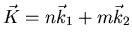can be written in terms of the real lattice vectors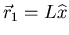and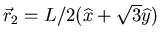so that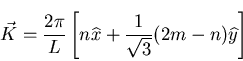(37)

where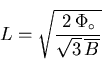(38)

is the distance between adjacent vortices. Combining Eqs. (3.36) and (3.37) gives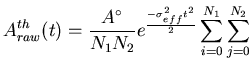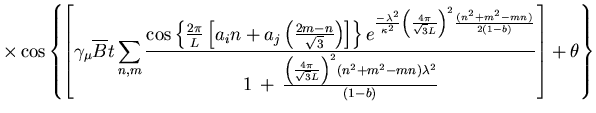(39)

where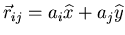is a vector in real space and the sum over ai and aj extends over a single unit cell. Eq. (3.39) can be substituted into Eq. (3.30) and the resulting theoretical corrected asymmetry used to fit the measured asymmetry.Next: 4-Counter Geometry and the Complex Polarization Up: Measuring the penetration depth with TF-SR Previous: The Relaxation Function
Jess H. Brewer
2001-09-28